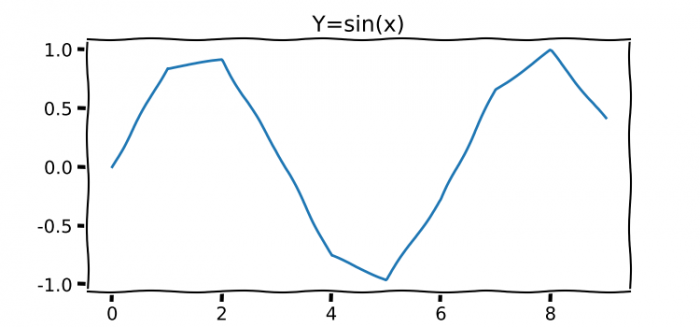# How to get XKCD font working in Matplotlib?

To get xkcd font working, we can use plt.xkcd() to turn on sketch-style drawing mode.

## Steps

• Set the figure size and adjust the padding between and around the subplots.
• Create x and y data points using numpy.
• Use plt.xkcd() to turn on sketch-style drawing mode.
• Create a new figure or activate an existing figure.
• Add an axis to the figure as part of a subplot arrangement.
• Plot x and y data points using plot() method.
• Place a text and title on the plot.
• To display the figure, use show() method.

## Example

from matplotlib import pyplot as plt
import numpy as np

plt.rcParams["figure.figsize"] = [7.50, 3.50]
plt.rcParams["figure.autolayout"] = True

x = np.arange(10)
y = np.sin(x)

plt.xkcd()

fig = plt.figure()

ax.plot(x, y)
ax.text(4, 4, '(4, 4)', size=16)

plt.title('Y=sin(x)')

plt.show()

## OutputUpdated on: 07-Jul-2021

563 Views